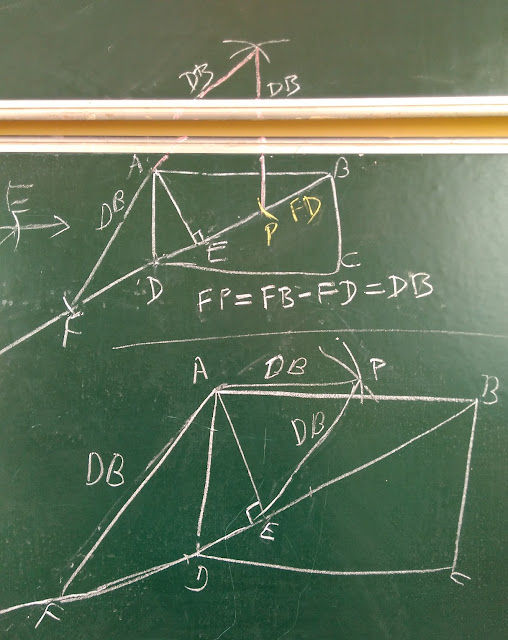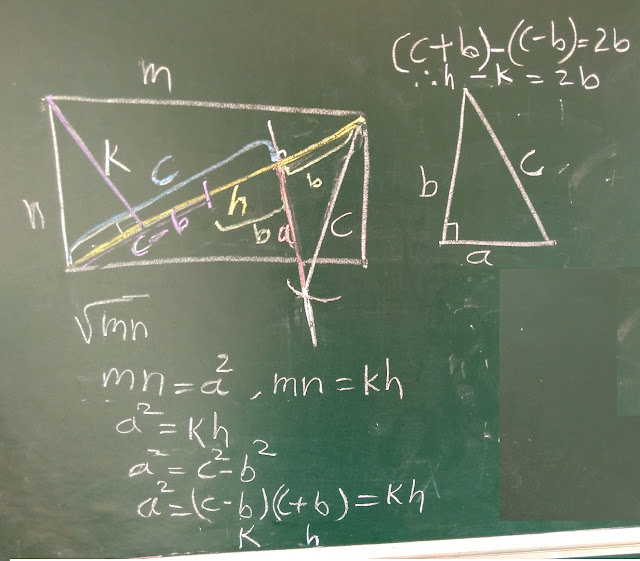## Monday, August 6, 2018

### Square of the size of Rectangle - PART-2

In my previous post, I had shared two methods figured out by students for Squaring the given Rectangle (area unchanged) with only compass & straight edge.

http://rupeshgesota.blogspot.com/2018/08/square-of-size-of-rectangle.html

And If you remember I had also mentioned in the end of the post that -

I had also posted this problem to another student over whatsapp and after almost a day-long struggle, she too sent me an interesting solution. I will share her solution in the Part-2 of this post along with another interesting variation of this problem, thanks to this student.

So here we go.... This was her solution:

As you can see, she has used the knowledge of generation of Pythagorean Triplets to find the square of required length i.e. sqrt(l x b) to get the area same as that of rectangle with sides l and b.

Perfect !!

I challenged her further -

What if you didn't know how to generate Triplets??

I knew that she had already persevered to get this result, but still it was important for her to know that there can be more methods...

Meanwhile, before Squaring the rectangle, she messaged me saying --

"I have converted the Rectangle into Rhombus... But still working on Squaring it.. "

This was her method:

Oh !! And this stimulated me to pose this (new) problem to my students over here now, who had solved the Squaring problem.

So when I did this the next day, it didn't take much time for them to crack it --

Vaishnavi had solved it the same way as above:Method-1

And this is how a group of three students had solved it, working together:Method-2

One of them came fwd to explain -

1) Extend side AB of Rectangle ABCD to get line l.
2) With center as C & radius = CD, get a point E on this line
3) Get F such that EF = EC
4) Join ED
5) So DCEF is Rhombus because all sides are equal.

How would you respond if your students give this explanation to you?

So I asked them to prove why DE = other 3 sides?

It was interesting to note that almost the whole class roared at me when I asked this question.

"Sir,.... It will be equal.... It's so obvious..."

" Not obvious to me.... Please prove it !"

So, you too may pause now -- and think for a while, as to how will you prove it?
-
-
-
-
-
-
-
-
-
-
-

So I, in my mind, had done this way --

"because one pair of opposite sides of quadr are equal and parallel, so its a parallelogram and since its one pair of adj sides are equal, so the parallelogram is a rhombus"

But, as I had suspected and as usual, they did this in a different way:

They proved the two triangles AFD and BFC are congruent by SSS test and hence by c.s.c.t. FD and EC are equal.

Well done !!

So I asked them if anyone has tried differently?

Kanchan came forward. This was her stroke --Method-3

She showed her work in the notebook to me first, which was perfect. But while explaining it to others on the board, she forgot a part of procedure and goofed up in between as you can see in the Lower diagram. Later when questioned for veracity, she could recollect and constructed the Upper diagram. This is how she explained --

1) Draw the diagonal and altitude of rectangle ABCD.
2) Extend BD such that you get AF = DB
3) From points A and E, make equal arcs of length DB to intersect at P.
4) AFEP is Rhombus.

What do you feel? :)

So when I asked her how EF equals other 3 sides, she realized the flaw in her explanation, and she corrected it in the figure above it.

1) & 2) same as above
3) She obtained side of length BD on the extended diagonal. But its interesting to see how she saw and achieved this. An easier way would be to make an arc of radius BD with F as center on FB.... But instead, she subtracted FD from FB to get the point P so that FP = BD

Interesting, isn't it?  :-)

Further steps were same as above...

So then I asked them, what's the similarity and differences in these 3 methods?
And they could answer this very well...

1) Can you answer this question precisely?
2) How would you / your students solve this problem?

Will be happy to know your views/ responses on this --

----
Thanks and Regards
Rupesh Gesota

PS: These students are from grade-8 and 9 Marathi medium government school based at Navi-Mumbai and are part of a maths enrichment program- MENTOR. To know more, check www.supportmentor.weebly.com

## Saturday, August 4, 2018

### Square of the size of Rectangle

This problem caught my attention while I was looking for something related to another geometrical problem on internet. And after studying it for a while, I was pretty sure that my students would love even this one.

"Given a rectangle of some size m x n, construct a square of area same as that of rectangle using only compass and a straight edge (not a marked ruler)"

To this, one of my students remarked -- "Sir, not even pencil?"  :-))

So I allowed them to struggle for some time (about 15 mins). Then I asked them if they had any ideas..

"Sir, we need a square of side = square-root of (m x n)."

Okay... So how do you get that?

"Do you remember some time back we had arrived at such term -----   sqrt(ab)"

After a while, one of them replied -- "Yes, in GM (geometric mean)."

I saw some students flipping back their pages after hearing this. But soon, they were stumped again.  I intervened again after @ 5 mins -

While few of them were still wondering, I could see some playing with their old friend - Pythagoras :)

When there was a road-block again after some time, I shot the final arrow --

Remember, we had done some exploration with the altitude of right triangle....

To this, couple of them just sprang up and started sketching again... and in no time, one of them shouted --

"Sir, I got the GM....!"

I could also see a pinch of embarrassment in his smiles... He had realized that we had worked on this in the past and he had forgotten this....

That in a right triangle, the length of the altitude to hypotenuse equals the square root of product of lengths of two segments into which the hypotenuse is divided...

We had arrived at this result while proving Pythagoras theorem using Similarity.
Never mind, who remembers things if they are not used often?

So now, he was happy that the side of the square was ready... But was stumped with the next step.... that to get this side, you need a Right Triangle... And how do you get that?

I noticed that while some students where listening to our conversation, there were few who were engrossed in their own work.... And one of them was Kanchan... She came with her piece of work after few more minutes. This is what she had done.Kanchan's work

For a teacher having some other (simpler) solution in his mind, it took an effort to not get overwhelmed by the size of such a work :-)

Let me illustrate her idea in following steps:

1) She made the diagonal of the given rectangle to get a Right Triangle. Draw its altitude (k)
to the hypotenuse (h)
2) Area of Rectangle = Area of Square = m x n. So a Square of side = sqrt(mn) is reqd.
3) Let area of such a square = m x n = a^2 (say) , where a is some +ve number = sqrt (mn)
4) Now the Right Triangle is Half the Rectangle.
So its Area = 1/2 x k  x  h  =  1/2  x  m  x n  which means, k x h = m x n
5) So a^2 = k x h
6) Now she makes this square of area a^2 sit on the side of another Right Triangle a, b, c.
Thus a^2 = c^2 - b^2  (by Pythagoras Thrm)
Thus a^2 = (c+b) (c-b) which is also equal to k x h.
7) Equating (c+b) to h and (c-b) to k
8) Now, h - k = (c+b) - (c-b) = 2b
9) So now perform this h - k    means with radius = k and center as bottom-left corner of rectangle, cut an arc on 'h' to divide it into two lengths:  the upper portion is 2b
10) Draw the perpendicular bisector of 2b to get 'b'.
11)  Now, since h = c + b, the lower part of h with b removed will be 'c'.
12) With this 'c' as radius and top-right corner of rectangle as center (i.e. b as base), cut an arc on the perpendicular bisector to get 'a'  (i.e. we construct the Right Triangle a-b-c)
13) And Hurray! We can now construct a square on this side 'a'. which will have same area as that of rectangle because a^2 = m x n ...... (from (3))

Fantastic !! Isn't it ??
(the color chalks were used by me later while taking the snaps -- for easier explanation)

So now I told her to actually do this construction. And she happily got engrossed into this.

Meanwhile, I found that Rohit had teamed up with 2 other students to work out the solution together. He was still wondering as to how to get the right angled Vertex at the top so that he can form a Right Triangle.

And one of his peers - Sahil - was just trying to draw circles with the foot of altitude as center and 'm' as radius and then 'n' as radius. They soon realized that this was not working.

When I noticed that they had persevered enough, I decided to give them a small hint --

And no wonder, in next couple of minutes, they declared, with a wide and relaxed smile on their face -- "Yes Sir, we are done! "

To this, Sahil taunted to Rohit -- "Because of my mistake!"      :-)  :-)  :-)

It was clearly evident that they knew what they have done, however the ever-doubting teacher in me couldn't resist himself & probed them for the explanation behind this...

They said it correctly...

1) Can you tell what property helped them to crack this problem eventually?
2) What are your views about the two methods, the investigation process and esp. the approach invented by Kanchan?